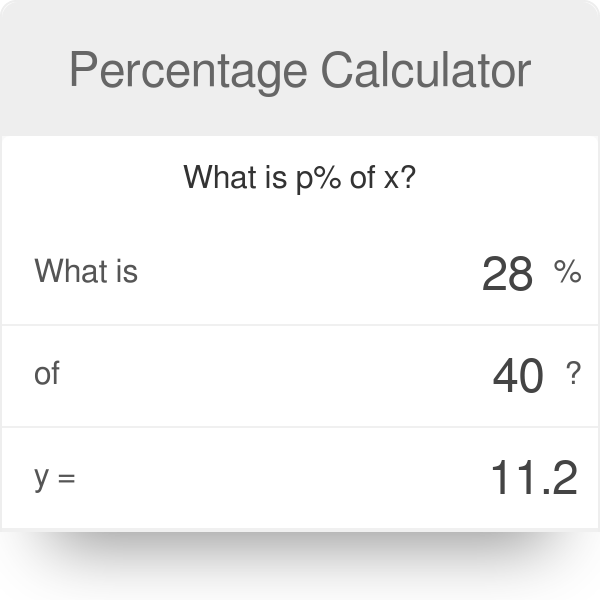# Percentage Calculator

Percent Meaning in Math – A percentage is a dimension number, and it is the ratio that defines as a fraction of 100. The percentage symbol is % or pct or pc. Normally it’s used to define the proportionate part of any number. Like 65% can be written like 65/100 or 65:100 or 0.65

## What is Percentage Calculator

Percentage Calculator is a free online tool that calculates the percentage of two numbers like 33 is the percentage of 100. The percentage sign is %.

## Percentage Calculator

Percentage (%) is the ratio or particular number that defines a fraction calculation of 100. The Symbol of percentage is % or pct or a percentage like 35%.

## How Percentage is Calculated

To calculate the percentage, divide the value by the total value and multiply the result by 100
Percentage formula – (Value/Total value) × 100
Example: 2/5 × 100 = 0.4 × 100 = 40 per cent

## Percentage Formula

In percentage, the formula is the algebraic question, that has 3 valuesP × V1 = V2

P – Percentage

V1 – First Value

V2 – Result on V1

Our percentage Calculator Provide automatic result for input values

P = 1.5/30 = 0.05 × 100 = 5%

## Percentage Increase Calculate

The Percentage Increase Calculator calculates the increasing differences from one value to another value in terms of % Percentage.You have to enter two values one is starting value and another is the final value to find an increasing percentage

### How to Calculate Percentage Increase

The percentage Increase Formula is

Percent increase = [(new value – original value)/original value] * 100

• Final Value minus starting value
• Divide that absolute amount value by the starting value
• Multiply the given value by 100 to get a percent increase
• It will find a positive or negative value if the result is positive then its percentage Increase otherwise its percentage decrease

## Percentage Decrease Calculate

The Percentage decrease Calculator calculates the negative difference from one value to another in the form of a percentage. You have to enter starting value and final value to get the percentage decrease

### How to Calculate Percentage Decrease

The percentage Decrease Formula is

Percent decrease = [(original value – new value)/original value] * 100

• First value minus the second value
• Divide the result by the absolute value of the starting value
• Multiply by 100 to get a percentage decrease
• If the percentage is negative it means that is an increased value